# Important Questions with Solutions for CBSE Class 9 Maths Chapter 12-Heron's Formula

Important questions of CBSE Class 9 Maths Chapter 12 Heron’s formula are provided here along with detailed solutions. Students who are preparing for their final exams can practise these questions to get better marks. All the problems are based on NCERT book and CBSE syllabus, presented by our Maths experts. Solving these important questions is the best way to ace the exams. Students can face the exams without fear.

Reach us at BYJU’S to get the important questions for CBSE Class 9 Maths all chapters with answers and attempt to get excellent marks in exams. Do the revision with the extra questions provided here.

## Important Questions & Answers For Class 9 Maths Chapter 12

Q.1: Find the area of a triangle whose two sides are 18 cm and 10 cm and the perimeter is 42cm.

Solution:

Assume that the third side of the triangle to be “x”.

Now, the three sides of the triangle are 18 cm, 10 cm, and “x” cm

It is given that the perimeter of the triangle = 42cm

So, x = 42 – (18 + 10) cm = 14 cm

∴ The semi perimeter of triangle = 42/2 = 21 cm

Using Heron’s formula,

Area of the triangle,

= √[s (s-a) (s-b) (s-c)]

= √[21(21 – 18) (21 – 10) (21 – 14)] cm2

= √[21 × 3 × 11 × 7] m2

= 21√11 cm2

Q.2: The sides of a triangle are in the ratio of 12: 17: 25 and its perimeter is 540cm. Find its area.

Solution:

The ratio of the sides of the triangle is given as 12: 17: 25

Now, let the common ratio between the sides of the triangle be “x”

∴ The sides are 12x, 17x and 25x

It is also given that the perimeter of the triangle = 540 cm

12x + 17x + 25x = 540 cm

=> 54x = 540cm

So, x = 10

Now, the sides of the triangle are 120 cm, 170 cm, 250 cm.

So, the semi perimeter of the triangle (s) = 540/2 = 270 cm

Using Heron’s formula,

Area of the triangle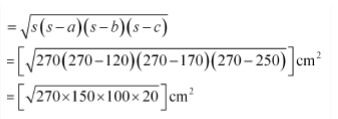= 9000 cm2

Q.3: A field is in the shape of a trapezium whose parallel sides are 25 m and 10 m. The non-parallel sides are 14 m and 13 m. Find the area of the field.

Solution:

First, draw a line segment BE parallel to the line AD. Then, from B, draw a perpendicular on the line segment CD.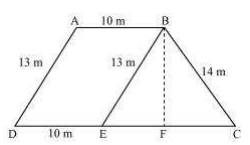Now, it can be seen that the quadrilateral ABED is a parallelogram. So,

AB = ED = 10 m

AD = BE = 13 m

EC = 25 – ED = 25 – 10 = 15 m

Now, consider the triangle BEC,

Its semi perimeter (s) = (13+ 14 + 15)/2 = 21 m

By using Heron’s formula,

Area of ΔBEC =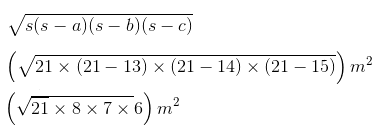= 84 m2

We also know that the area of ΔBEC = (½) × CE × BF

84 cm2 = (½) × 15 × BF

=> BF = (168/15) cm = 11.2 cm

So, the total area of ABED will be BF × DE, i.e. 11.2 × 10 = 112 m2

∴ Area of the field = 84 + 112 = 196 m2

Q.4: A rhombus-shaped field has green grass for 18 cows to graze. If each side of the rhombus is 30 m and its longer diagonal is 48 m, how much area of grass field will each cow be getting?

Solution:

Draw a rhombus-shaped field first with the vertices as ABCD. The diagonal AC divides the rhombus into two congruent triangles which are having equal areas. The diagram is as follows.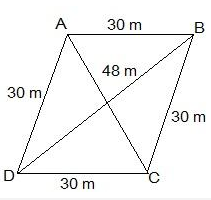Consider the triangle BCD,

Its semi-perimeter = (48 + 30 + 30)/2 m = 54 m

Using Heron’s formula,

Area of the ΔBCD =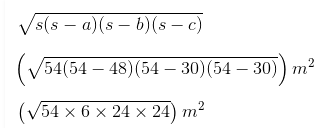= 432 m2

∴ Area of field = 2 × area of the ΔBCD = (2 × 432) m2 = 864 m2

Thus, the area of the grass field that each cow will be getting = (864/18) m2 = 48 m2

Q.5: Find the cost of laying grass in a triangular field of sides 50 m, 65 m and 65 m at the rate of Rs 7 per m2.

Solution:

According to the question,

Sides of the triangular field are 50 m, 65 m and 65 m.

Cost of laying grass in a triangular field = Rs 7 per m2

Let a = 50, b = 65, c = 65

s = (a + b + c)/2

⇒ s = (50 + 65 + 65)/2

= 180/2

= 90.

Area of triangle = √(s(s-a)(s-b)(s-c))

= √(90(90-50)(90-65)(90-65))

= √(90×40×25×25)

= 1500m2

Cost of laying grass = Area of triangle ×Cost per m2

= 1500×7

= Rs.10500

Q.6: The perimeter of an isosceles triangle is 32 cm. The ratio of the equal side to its base is 3: 2. Find the area of the triangle.

Solution:

According to the question,

The perimeter of the isosceles triangle = 32 cm

It is also given that,

Ratio of equal side to base = 3 : 2

Let the equal side = 3x

So, base = 2x

Perimeter of the triangle = 32

⇒ 3x + 3x + 2x = 32

⇒ 8x = 32

⇒ x = 4.

Equal side = 3x = 3×4 = 12

Base = 2x = 2×4 = 8

The sides of the triangle = 12cm, 12cm and 8cm.

Let a = 12, b = 12, c = 8

s = (a + b + c)/2

⇒ s = (12 + 12 + 8)/2

= 32/2

= 16.

Area of the triangle = √(s(s-a)(s-b)(s-c))

= √(16(16-12)(16-12)(16-8))

= √(16×4×4×8)

= 32√2 cm2

Q.7: How much paper of each shade is needed to make a kite given in the figure, in which ABCD is a square with diagonal 44 cm.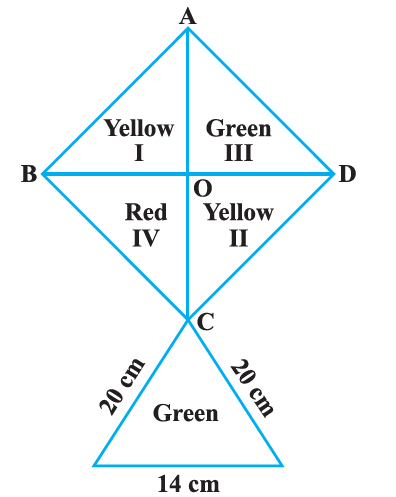Solution:

According to the figure,

AC = BD = 44cm

AO = 44/2 = 22cm

BO = 44/2 = 22cm

From ΔAOB,

AB2 = AO2 + BO2

⇒ AB2 = 222 + 222

⇒ AB2 = 2 × 222

⇒ AB = 22√2 cm

Area of square = (Side)2

= (22√2)2

= 968 cm2

Area of each triangle (I, II, III, IV) = Area of square /4

= 968 /4

= 242 cm2

To find area of lower triangle,

Let a = 20, b = 20, c = 14

s = (a + b + c)/2

⇒ s = (20 + 20 + 14)/2 = 54/2 = 27.

Area of the triangle = √[s(s-a)(s-b)(s-c)]

= √[27(27-20)(27-20)(27-14)]

= √[27×7×7×13]

= 131.14 cm2

Therefore,

We get,

Area of Red = Area of IV

= 242 cm2

Area of Yellow = Area of I + Area of II

= 242 + 242

= 484 cm2

Area of Green = Area of III + Area of the lower triangle

= 242 + 131.14

= 373.14 cm2

Q.8: A rectangular plot is given for constructing a house, having a measurement of 40 m long and 15 m in the front. According to the laws, a minimum of 3 m, wide space should be left in the front and back each and 2 m wide space on each of other sides. Find the largest area where a house can be constructed.

Solution: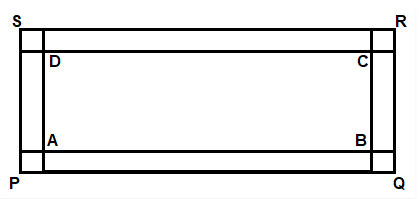Let the given rectangle be rectangle PQRS,

According to the question,

PQ = 40m and QR = 15m

As 3m is left in both front and back,

AB = PQ -3 -3

⇒ AB = 40 -6

⇒ AB = 34m

Also,

Given that 2m has to be left at both the sides,

BC = QR -2 – 2

⇒ BC = 15 -4

⇒ BC = 11m

Now, Area left for house construction is the area of ABCD.

Hence,

Area(ABCD) = AB × CD

= 34 × 11

= 374 m2

### Extra Questions for Class 9 Maths Chapter 12 (Heron’s Formula)

1. A field in the form of the parallelogram has sides 60 m and 40 m, and one of its diagonals is 80m long. Find the area of the parallelogram.
2. The sides of a quadrilateral ABCD are 6cm, 8cm, 12cm and 14cm respectively. The angle between the first two sides is a right angle. Find its area.
3. A rhombus-shaped sheet with perimeter 40cm and one diagonal 12 cm, is painted on both sides at the rate of Rs. 5 per m2. Find the cost of painting.
4. From a point in the interior of an equilateral triangle, perpendicular is drawn on the three sides. The lengths of the perpendicular are 14cm, 10cm and 6cm. Find the area of the triangle.
5. The perimeter of a triangular field is 240m with two sides 78m and 50m. Now, calculate the length of the altitude on the side of 50m length from its opposite vertex.